## Solar Storms Could Knock Out Internet For Months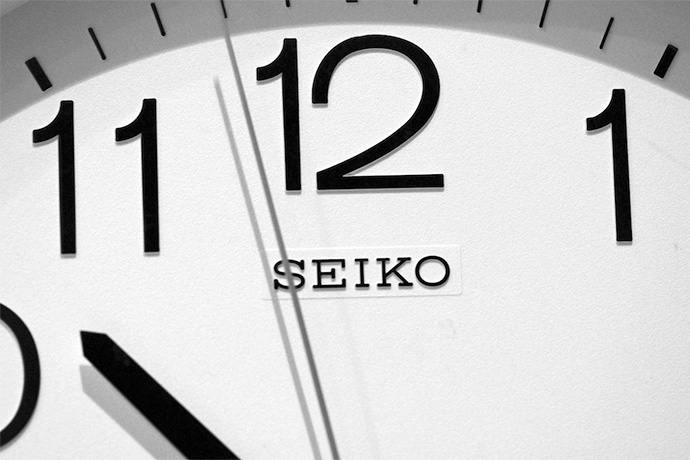## How Long is a Second?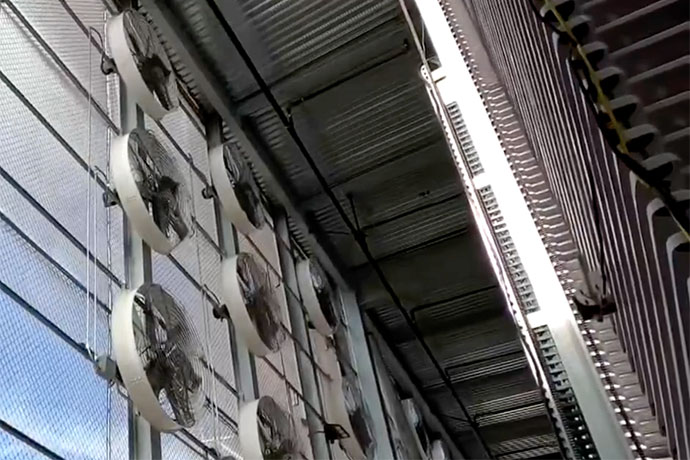## First US Carbon Capture Plant Opens#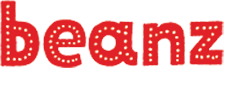dark mode light mode Search Menu

# Binary Riddles

Personal Creations on Flickr

Look at the following list of numbers. Do you notice a pattern, from left to right, that creates the next number in this sequence?

1, 2, 4, 8

What number do you think comes next? If you guessed 16, you have an idea how binary numbers work. Binary numbers have two digits, either a 0 or a 1. The position of the zeroes and ones determines the value of each 0 or 1.

For example, 1101 is a binary number. The values of each 0 and 1 depends on where it is located, counting from the right hand number.

8 4 2 1 –> Value based on position, right to left
1 1 0 1 –> 0 or 1 in position, right to left

In this example, to determine the value of 1101, we would add up the values based on the position of each 1 digit. There is a 1 in the 8, 4, and 1 value positions. Because 8 + 4 + 1 = 13, the value of 1101 is 13.

Adding up numbers from this list – 1, 2, 4, 8, 16 – we can make any number from one to thirty one. Why 31? Add 16 + 8 + 4 + 2 + 1 in our list! Can you guess the binary number for 31? It’s a 1 in every position counted right to left: 11111 or 16 + 8 + 4 + 2 + 1.

Take a look at the chart below:

Chart A

 16 8 4 0 1 <-- Values 0 1 1 0 1 Total: 13

If we add up the value above each cell with a 1 in it we get 13 (8+4+1).

Let’s try another one. Add up the values above each box with a 1 below.

Chart B

 16 8 4 2 0 <-- Values 1 1 1 1 0 Total: 30

If you got 30, you’re right!

Although computers perform all sorts of complex tasks, a computer only knows whether or not electricity is passing through part of a circuit within it. The cells with a 1 represent where there is electricity, and the cells with 0 represent where there is none. Take a look at the updated chart below. This time each shaded box is marked with a 1 and each blank box with a 0.

 16 8 4 2 1 <-- Values 0 1 0 0 1 Total: 9

In binary, the number 9 (8 +1) is represented by 1001. The leading zero is not written as it simply indicates that no number beyond eight was used in the calculation. If we do the same for the number 30, we will find that it’s binary representation is 11110 (16 + 8 + 4 + 2), reading and counting from left to right..

For some extra binary fun, challenge yourself to decode the binary boxes below in order to answer a few riddles.

Instructions: Each row of each table represents a number in binary form, using zeros and ones. Add up the numbers in the top row that correspond to each shaded box. Find the sum in the key at the bottom of the page, and replace it with the corresponding letter to decode the answer to each riddle.

I am an odd number. Take away a letter and I become even. What number am I?

Hint: The first row of the table has the 16, 2, and 1 columns with a 1. Adding these up we get 19 (16 + 2 + 1). In the key below, we see that 19 corresponds to the letter “s”. So the first letter of the answer is “s”. The second row has the 4 and 1 columns have a 1 for a sum of 5 (“e”), and so on.

I’m tall when I’m young, and I’m short when I’m old, what am I? Note: The blackened row indicates a space between two words.

You see me once in June, twice in November, but not at all in May. What am I?

If you fill in each shaded box in the charts above with a one and each blank box with a zero, you will see how each sum is represented in standard binary form!

#### Binary in Beanz

https://kidscodecs.com/binary-numbers/

#### Binary from TED Ed

https://ed.ted.com/lessons/how-exactly-does-binary-code-work-jose-americo-n-l-f-freitas

#### Binary numbers

https://kids.kiddle.co/Binary_number

#### Binary Number system

https://www.mathsisfun.com/binary-number-system.html

#### Teaching kids binary

https://www.sciencekiddo.com/teach-kids-binary/

#### Binary code necklaces

##### Related Posts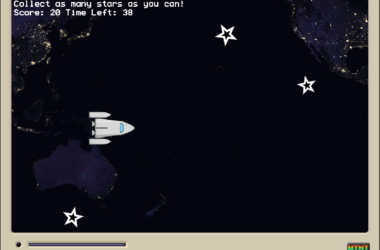## Make a Mini Micro Collection Game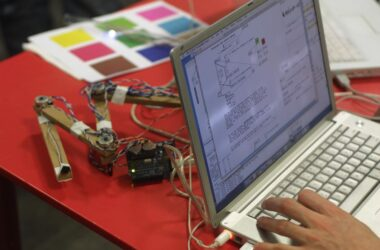## Geeking Out with PureData Music## Minecraft Makeover